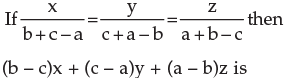Courses

#a)1b)0c)5d)none of theseCorrect answer is option 'B'. Can you explain this answer? Related Test: Test: Ratio And Proportion, Indices, Logarithms - 2

## CA Foundation Question

By Kavya C · May 25, 2020 ·CA Foundation
Mini Dharsini answered May 25, 2020
(b-c)x=(b-c)(b+c-a). ,(c-a)y=(c-a)(c+a-b). ,(a-b)z=(a-b)(a+b-c)

=( b^2+ bc-ab-bc-c^2+ac) + (c ^2+ac-bc-ac-a^2+ab) +
(a^2+ab-ac-ab-b^2+bc).
= 0

Anjali Verma answered Sep 25, 2018
Can anyone solve it for me

Ayush Agarwal answered Jul 23, 2020
bcus the question is based on tricks of cyclic order

Latha Gone answered Jul 23, 2020
Let X=(b+c-a);y=(c+a-b);z=(a+b-c)

=(b-c)(b+c-a)+(c-a)(c+a-b)+(a-b)(a+b-c)

=b^2-c^2-ab+ac+c^2-a^2-bc+ab+a^2-b^2-ac+bc

=0

Baby Devi answered May 25, 2020
0